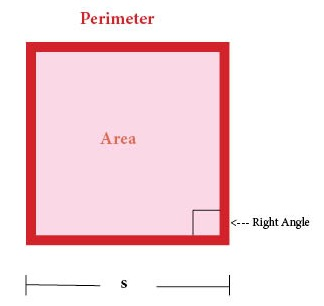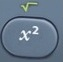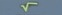## Square

Square = Flat Shape with Four Equal Sides (with four right angles)Basics
s = Side
P = Perimeter = distance along sides of square
A = Area = area covering surface of square (measured in square units)
Right Angle = 90°

A square has four right angles.
The opposite sides of a square are parallel.
A square is a special form of a parallelogram in which all angles are right angles and all sides are equal.

The math involving the geometric square often requires the calculation of arithmetic squares and square roots.is the squaring command.is the square root command.activatesabove.

Formulas (provided by Mathematics Formula Sheet)

P =
4s
A = s²

Formulas (NOT provided by Mathematics Formula Sheet)

s = P ÷ 4
s = A

Question
The side of a square postage stamp is 2.5 centimeters.  What is its perimeter?

10 centimeters

P = 4s
P = 4 × 2.5 = 10

Calculator Click What You See Commentblinker clears screen
4 × 2.5 4*2.5 P = 4s10 Answer
###### Square

Question
The side of a square postage stamp is 2.5 centimeters.  What is its area?

6.25 square centimeters

A = s²
A = 2.5² = 6.25

Calculator Click What You See Commentblinker clears screen
2.52.5² A = s²6.25 Answer
###### Square

Question
The perimeter of a square postage stamp is 14 centimeters.  How long is its side?

3.5 centimeters

s = P ÷ 4
s = 14 ÷ 4 = 3.5

Calculator Click What You See Commentblinker clears screen
14 ÷ 4 14÷4 s = P ÷ 43.5 Answer
###### Square

Question
The area of a square postage stamp is 13.69 square centimeters.  How long is its side?

3.7 centimeters

s = A
s = 13.69 = 3.7

Calculator Click What You See Commentblinker clears screen13.69 13.69 s = A3.7 Answer
###### Square

Question
The perimeter of a square postage stamp is 18.2 centimeters.  What is its area?

20.7025 square centimeters

s = P ÷ 4
s = 18.2 ÷ 4 = 4.55

A = s²
A = 4.55² = 20.7025

Calculator Click What You See Commentblinker clears screen
18.2 ÷ 4 18.2÷4 s = P ÷ 44.55 s
4.554.55² A = s²20.7025 Answer
###### Square

Question
The area of a square postage stamp is 7.29 square centimeters.  What is its perimeter?

10.8 centimeters

s = A
s = 7.29 = 2.7

P = 4s
P = 4 × 2.7 = 10.8

Calculator Click What You See Commentblinker clears screen7.29 7.29 s = A2.7 s
4 × 2.7 4*2.7 P = 4s10.8 Answer
###### Square

Practice – Questions
1.  The side of a square tile is 6 inches.  What is its perimeter?

2.  The side of a square tile is 6 inches.  What is its area?

3.  The perimeter of a square tile is 49.6 inches.  What is its area?

4.  The area of a square tile is 128.5956 square inches.  What is its perimeter?

5.  With reference to Question 4, round your answer to the nearest tenth.